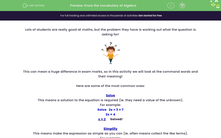# Know the Vocabulary of Algebra

In this worksheet, students will interpret and apply mathematical command wordsKey stage:  KS 3

Curriculum topic:   Algebra

Curriculum subtopic:   Use and Interpret Algebraic Notation

Difficulty level:#### Worksheet Overview

Lots of students are really good at maths, but the problem they have is working out what the question is asking for!This can mean a huge difference in exam marks, so in this activity we will look at the command words and their meaning!

Here are some of the most common ones:

Solve

This means a solution to the equation is required (ie. they need a value of the unknown).

For example:

Solve   2x + 3 = 7

2x = 4

x = 2      Solved!

Simplify

This means make the expression as simple as you can (ie. often means collect the like terms).

For example:

Simplify   2c + 4v + 3c + 5v

= 5c + 9v     Simplified!

Expand

This means remove any brackets.

For example:

Expand    2(3x + 5)            ( 2x3x = 6x    and 2 x 5 = 10)

= 6x + 10     Expanded!

Factorise

This means insert brackets by taking out common factors.

For example:

Factorise 2w + 6

2(w + 3)       Factorised!

Does that all make sense?Let's practise these command words.

### What is EdPlace?

We're your National Curriculum aligned online education content provider helping each child succeed in English, maths and science from year 1 to GCSE. With an EdPlace account you’ll be able to track and measure progress, helping each child achieve their best. We build confidence and attainment by personalising each child’s learning at a level that suits them.

Get started